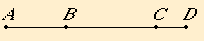# Proposition 80

To a first apotome of a medial straight line only one medial straight line can be annexed which is commensurable with the whole in square only and which contains with the whole a rational rectangle.
X.74

Let AB be a first apotome of a medial straight line, and let KC be an annex to AB. Then AC and CB are medial straight lines commensurable in square only such that the rectangle AC by CB which they contain is rational.

I say that no other medial straight line can be annexed to AB which is commensurable with the whole in square only and which contains with the whole a rational area.X.74

If possible, let DB also be so annexed. Then AD and DB are medial straight lines commensurable in square only such that the rectangle AD by DB which they contain is rational.

II.7

Now, since the excess of the sum of the squares on AD and DB over twice the rectangle AD by DB is also the excess of the sum of the squares on AC and CB over twice the rectangle AC by CB, for they exceed by the same, the square on AB, therefore, alternately, the excess of the sum of the squares on AD and DB over the sum of the squares on AC and CB is also the excess of twice the rectangle AD by DB over twice the rectangle AC by CB.

But twice the rectangle AD by DB exceeds twice the rectangle AC by CB by a rational area, for both are rational.

Therefore the sum of the squares on AD and DB also exceeds the sum of the squares on AC and CB by a rational area, which is impossible, for both are medial, and a medial area does not exceed a medial by a rational area.

Therefore, to a first apotome of a medial straight line only one medial straight line can be annexed which is commensurable with the whole in square only and which contains with the whole a rational rectangle.

Q.E.D.

## Guide

This proposition is not used in the rest of the Elements.